1. /
2. CBSE
3. /
4. Class 10
5. /
6. Mathematics
7. /
8. Class 10 Maths Sample...

# Class 10 Maths Sample Papers 2022-23### myCBSEguide App

Download the app to get CBSE Sample Papers 2023-24, NCERT Solutions (Revised), Most Important Questions, Previous Year Question Bank, Mock Tests, and Detailed Notes.

CBSE has two courses in class 10 Maths. These are Maths Basic and Maths Standard. You can get CBSE Class 10 Maths Sample Papers 2022-23 from myCBSEguide for both Basic and Standard courses. We have designed them as per the latest marking scheme and blueprint issued for the session 2022-23.

## Sample Paper of Maths Class 10 –  PDF with Solution

We are providing class 10 Maths Sample Papers 2022-23 as per the new marking scheme and the blueprint for Maths Basic and Maths Standard. Maths is a subject that demands more practice but it is a scoring subject as well. Sample papers give you an idea of the actual question paper and boost your confidence to score well. Sample Model test Papers for class 10 Maths with Solutions are available on the myCBSEguide app. Students can download these model papers and practice them at home.

### Maths Basic Sample Paper 2023 with Solution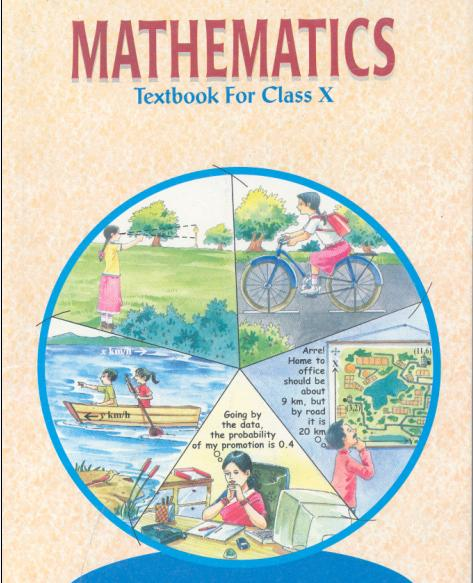## CBSE Class 10 Maths Sample Papers 2022-23

myCBSEguide provides CBSE Class 10 Sample Papers of Mathematics for the years 2022, 2023, 2024 with solutions in PDF format for free download. You may have noticed that the format of questions in class 10 maths sample papers 2023 is a bit different. You will get a very limited number of straightforward questions. There are a number of questions from real-life situations. Many questions are directly or indirectly evaluating your competencies. So, you need to undergo regular practice to get good marks in your exams.

## Model Papers Class 10 Maths Standard 2023

CBSE Class 10
Mathematics Standard (Code No. 041)
(Sample Paper 2022-23)

Time Allowed: 3 Hrs.
Maximum Marks: 80
General Instructions:

1. This Question Paper has 5 Sections A-E.
2. Section A has 20 MCQs carrying 1 mark each
3. Section B has 5 questions carrying 02 marks each.
4. Section C has 6 questions carrying 03 marks each.
5. Section D has 4 questions carrying 05 marks each.
6. Section E has 3 case based integrated units of assessment (04 marks each) with subparts of the values of 1, 1 and 2 marks each respectively.
7. All Questions are compulsory. However, an internal choice in 2 Qs of 5 marks, 2 Qs of 3 marks and 2 Questions of 2 marks has been provided. An internal choice has been provided in the 2marks questions of Section E
8. Draw neat figures wherever required. Take {tex}\pi{/tex} = 22/7 wherever required if not stated.

### Class 10 Maths Standard SECTION A

Section A consists of 20 questions of 1 mark each.

1. Let a and b be two positive integers such that a = p3q4 and b = p2q3, where p and q are prime numbers. If HCF(a, b) = pmqn and LCM(a, b) = prqs, then (m+n)(r+s)=
1. 15
2. 30
3. 35
4. 72
2. Let p be a prime number. The quadratic equation having its roots as factors of p is
1. x2 – px + q = 0
2. x2 – (p + 1)x + q = 0
3. x2 + (p + 1)x + p = 0
4. x2 – px + p + 1 = 0
3. If {tex}\alpha{/tex} and {tex}\beta{/tex} are the zeros of a polynomial f(x) = px2 – 2x + 3p and {tex}\alpha+\beta=\alpha \beta{/tex}, then p is
1. -2/3
2. 2/3
3. 1/3
4. -1/3
4. If the system of equations 3x + y = 1 and (2k – 1)x + (k – 1)y =2k + 1 is inconsistent, then k =
1. -1
2. 0
3. 1
4. 2
5. If the vertices of a parallelogram PQRS taken in order are P(3,4), Q(-2,3) and R(-3,-2), then the coordinates of its fourth vertex S are
1. (-2, -1)
2. (-2, -3)
3. (2, -1)
4. (1, 2)
6. {tex}\triangle \mathrm{ABC} \sim \triangle \mathrm{PQR}{/tex}. If AM and PN are altitudes of {tex}\triangle \mathrm{ABC}{/tex} and {tex}\triangle \mathrm{PQR}{/tex} respectively and AB: PQ2 = 4 : 9, then AM : PN =
1. 3 : 2
2. 16 : 81
3. 4 : 9
4. 2 : 3
7. If x tan 60o cos 60= sin60o cot 60o, then x =
1. cos30o
2. tan30o
3. sin30o
4. cot30o
8. If sin{tex}\theta{/tex} + cos{tex}\theta{/tex} = {tex}\sqrt{2}{/tex}, then tan{tex}\theta{/tex} + cot{tex}\theta{/tex} =
1. 1
2. 2
3. 3
4. 4
9. In the given figure, DE ∥ BC, AE = a units, EC = b units, DE = x units and BC = y units. Which of the following is true?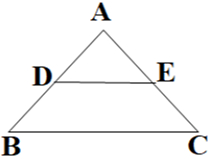1. {tex}\mathrm{x}=\frac{a+b}{a y}{/tex}
2. {tex}\mathrm{y}=\frac{a x}{a+b}{/tex}
3. {tex}\mathrm{x}=\frac{a y}{a+b}{/tex}
4. {tex}\frac{x}{y}=\frac{a}{b}{/tex}
10. ABCD is a trapezium with AD ∥ BC and AD = 4cm. If the diagonals AC and BD intersect each other at O such that AO/OC = DO/OB =1/2, then BC =
1. 6cm
2. 7cm
3. 8cm
4. 9cm
11. If two tangents inclined at an angle of 60o are drawn to a circle of radius 3cm, then the length of each tangent is equal to
1. {tex}\frac{3 \sqrt{3}}{2}{/tex}cm
2. 3cm
3. 6cm
4. {tex}3 \sqrt{3}{/tex}
12. The area of the circle that can be inscribed in a square of 6cm is
1. 36{tex}\pi{/tex} cm2
2. 18{tex}\pi{/tex} cm2
3. 12{tex}\pi{/tex} cm2
4. 9{tex}\pi{/tex} cm2
13. The sum of the length, breadth and height of a cuboid is {tex}6 \sqrt{3}{/tex} cm and the length of its diagonal is {tex}2 \sqrt{3}{/tex} cm. The total surface area of the cuboid is
1. 48 cm2
2. 72 cm2
3. 96 cm2
4. 108 cm2
14. If the difference of Mode and Median of a data is 24, then the difference of median and mean is
1. 8
2. 12
3. 24
4. 36
15. The number of revolutions made by a circular wheel of radius 0.25m in rolling a distance of 11km is
1. 2800
2. 4000
3. 5500
4. 7000
16. For the following distribution,
 Class 0-5 5-10 10-15 15-20 20-25 Frequency 10 15 12 20 9

the sum of the lower limits of the median and modal class is

1. 15
2. 25
3. 30
4. 35
17. Two dice are rolled simultaneously. What is the probability that 6 will come up at least once?
1. 1/6
2. 7/36
3. 11/36
4. 13/36
18. If 5 tan{tex}\beta{/tex} = 4, then {tex}\frac{5 \sin \beta-2 \cos \beta}{5 \sin \beta+2 \cos \beta}{/tex} =
1. 1/3
2. 2/5
3. 3/5
4. 6

DIRECTION: In the question number 19 and 20, a statement of Assertion (A) is followed by a statement of Reason (R). Choose the correct option

1. Statement A (Assertion): If product of two numbers is 5780 and their HCF is 17, then their LCM is 340
Statement R( Reason): HCF is always a factor of LCM

1. Both assertion (A) and reason (R) are true and reason (R) is the correct explanation of assertion (A)
2. Both assertion (A) and reason (R) are true and reason (R) is not the correct explanation of assertion (A)
3. Assertion (A) is true but reason (R) is false.
4. Assertion (A) is false but reason (R) is true.
2. Statement A (Assertion): If the co-ordinates of the mid-points of the sides AB and AC of {tex}\triangle{/tex}ABC are D(3, 5) and E(-3, -3) respectively, then BC = 20 units Statement R( Reason): The line joining the mid points of two sides of a triangle is parallel to the third side and equal to half of it.
1. Both assertion (A) and reason (R) are true and reason (R) is the correct explanation of assertion (A)
2. Both assertion (A) and reason (R) are true and reason (R) is not the correct explanation of assertion (A)
3. Assertion (A) is true but reason(R) is false.
4. Assertion (A) is false but reason(R) is true.

### Class 10 Maths Standard SECTION B

Section B consists of 5 questions of 2 marks each.

1. If 49x + 51y = 499, 51x + 49y = 501, then find the value of x and y
2. In the given figure below, {tex}\frac{\mathrm{AD}}{\mathrm{AE}}=\frac{\mathrm{AC}}{\mathrm{BD}}{/tex} and {tex}\angle 1=\angle 2 {/tex}. Show that {tex}\triangle \mathrm{BAE} \sim \triangle \mathrm{CAD}{/tex}.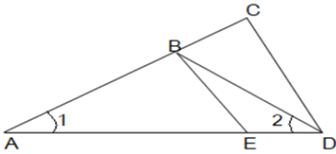3. In the given figure, O is the centre of circle. Find {tex}\angle{/tex}AQB, given that PA and PB are tangents to the circle and ∠APB= 75o.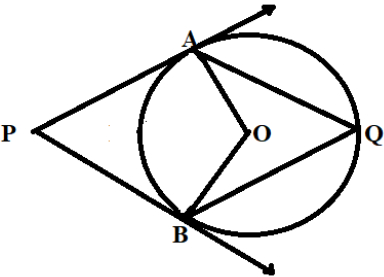4. The length of the minute hand of a clock is 6cm. Find the area swept by it when it moves from 7:05 p.m. to 7:40 p.m.

OR

In the given figure, arcs have been drawn of radius 7cm each with vertices A, B, C and D of quadrilateral ABCD as centres. Find the area of the shaded region.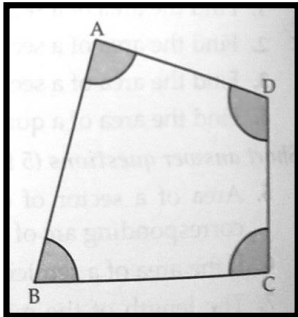5. If sin(A + B) = 1 and cos(A – B)= {tex}\sqrt{3 / 2}{/tex}, 0o < A + B {tex}\leq{/tex} 90o and A > B, then find the measures of angles A and B.

OR

Find an acute angle {tex}\theta{/tex} when {tex}\frac{\cos \theta-\sin \theta}{\cos \theta+\sin \theta}=\frac{1-\sqrt{3}}{1+\sqrt{3}}{/tex}

### Class 10 Maths Standard SECTION C

Section C consists of 6 questions of 3 marks each.

1. Given that {tex}\sqrt{3}{/tex} is irrational, prove that 5 + {tex}2 \sqrt{3}{/tex} is irrational.
2. If the zeroes of the polynomial x2 + px + q are double in value to the zeroes of the polynomial 2x2 – 5x – 3, then find the values of p and q.
3. A train covered a certain distance at a uniform speed. If the train would have been 6 km/h faster, it would have taken 4 hours less than the scheduled time. And, if the train were slower by 6 km/hr ; it would have taken 6 hours more than the scheduled time. Find the length of the journey.

OR

Anuj had some chocolates, and he divided them into two lots A and B. He sold the first lot at the rate of ₹ 2 for 3 chocolates and the second lot at the rate of ₹ 1 per chocolate, and got a total of ₹ 400. If he had sold the first lot at the rate of ₹ 1 per chocolate, and the second lot at the rate of ₹ 4 for 5 chocolates, his total collection would have been ₹ 460. Find the total number of chocolates he had.

4. Prove the following that-
{tex}\frac{\tan ^3 \theta}{1+\tan ^2 \theta}+\frac{\cot ^3 \theta}{1+\cot ^2 \theta}{/tex} = sec{tex}\theta{/tex} cosec{tex}\theta{/tex} – 2sin{tex}\theta{/tex} cos{tex}\theta{/tex}
5. Prove that a parallelogram circumscribing a circle is a rhombus

OR

In the figure XY and X’Y’ are two parallel tangents to a circle with centre O and another tangent AB with point of contact C interesting XY at A and X’Y’ at B, what is the measure of {tex}\angle{/tex}AOB.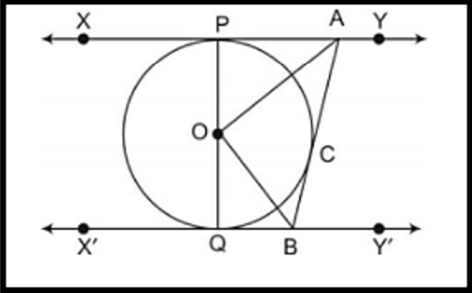6. Two coins are tossed simultaneously. What is the probability of getting
2. At most one tail?
3. A head and a tail?

### Class 10 Maths Standard SECTION D

Section D consists of 4 questions of 5 marks each.

1. To fill a swimming pool two pipes are used. If the pipe of larger diameter used for 4 hours and the pipe of smaller diameter for 9 hours, only half of the pool can be filled. Find, how long it would take for each pipe to fill the pool separately, if the pipe of smaller diameter takes 10 hours more than the pipe of larger diameter to fill the pool?

OR

In a flight of 600km, an aircraft was slowed down due to bad weather. Its average speed for the trip was reduced by 200 km/hr from its usual speed and the time of the flight increased by 30 min. Find the scheduled duration of the flight.

2. Prove that if a line is drawn parallel to one side of a triangle intersecting the other two sides in distinct points, then the other two sides are divided in the same ratio.
Using the above theorem prove that a line through the point of intersection of the diagonals and parallel to the base of the trapezium divides the non parallel sides in the same ratio.
3. Due to heavy floods in a state, thousands were rendered homeless. 50 schools collectively decided to provide place and the canvas for 1500 tents and share the whole expenditure equally. The lower part of each tent is cylindrical with base radius 2.8 m and height 3.5 m and the upper part is conical with the same base radius, but of height 2.1 m. If the canvas used to make the tents costs ₹ 120 per m2, find the amount shared by each school to set up the tents.

OR

There are two identical solid cubical boxes of side 7cm. From the top face of the first cube a hemisphere of diameter equal to the side of the cube is scooped out. This hemisphere is inverted and placed on the top of the second cube’s surface to form a dome. Find

1. the ratio of the total surface area of the two new solids formed
2. volume of each new solid formed.
4. The median of the following data is 525. Find the values of x and y, if the total frequency is 100
 Class interval Frequency 0-100 2 100-200 5 200-300 x 300-400 12 400-500 17 500-600 20 600-700 y 700-800 9 800-900 7 900-1000 4

### Class 10 Maths Standard SECTION E

Case study based questions are compulsory.

1. A tiling or tessellation of a flat surface is the covering of a plane using one or more geometric shapes, called tiles, with no overlaps and no gaps. Historically, tessellations were used in ancient Rome and in Islamic art. You may find tessellation patterns on floors, walls, paintings etc. Shown below is a tiled floor in the archaeological Museum of Seville, made using squares, triangles and hexagons.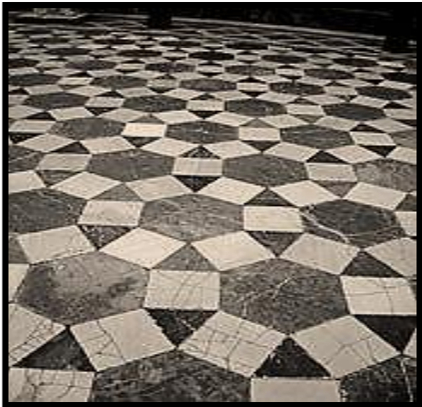A craftsman thought of making a floor pattern after being inspired by the above design. To ensure accuracy in his work, he made the pattern on the Cartesian plane. He used regular octagons, squares and triangles for his floor tessellation pattern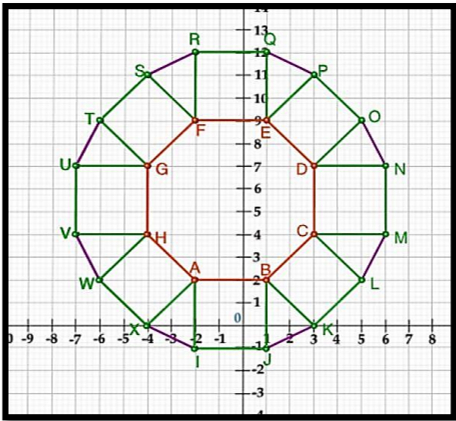Use the above figure to answer the questions that follow:

1. What is the length of the line segment joining points B and F?
2. The centre ‘Z’ of the figure will be the point of intersection of the diagonals of quadrilateral WXOP. Then what are the coordinates of Z?
3. What are the coordinates of the point on y axis equidistant from A and G?

OR

What is the area of area of Trapezium AFGH?

2. The school auditorium was to be constructed to accommodate at least 1500 people. The chairs are to be placed in concentric circular arrangement in such a way that each succeeding circular row has 10 seats more than the previous one.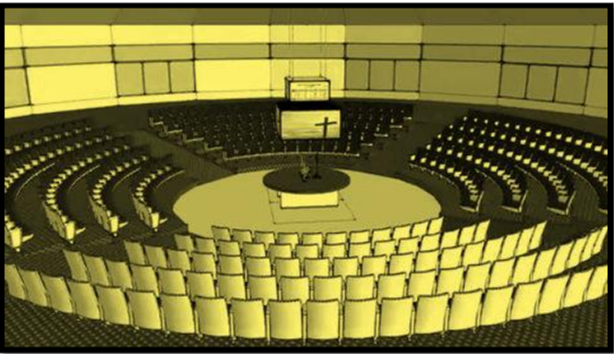1. If the first circular row has 30 seats, how many seats will be there in the 10th row?
2. For 1500 seats in the auditorium, how many rows need to be there?

OR

If 1500 seats are to be arranged in the auditorium, how many seats are still left to be put after 10th row?

3. If there were 17 rows in the auditorium, how many seats will be there in the middle row?
3. We all have seen the airplanes flying in the sky but might have not thought of how they actually reach the correct destination. Air Traffic Control (ATC) is a service provided by ground-based air traffic controllers who direct aircraft on the ground and through a given section of controlled airspace, and can provide advisory services to aircraft in non-controlled airspace. Actually, all this air traffic is managed and regulated by using various concepts based on coordinate geometry and trigonometry.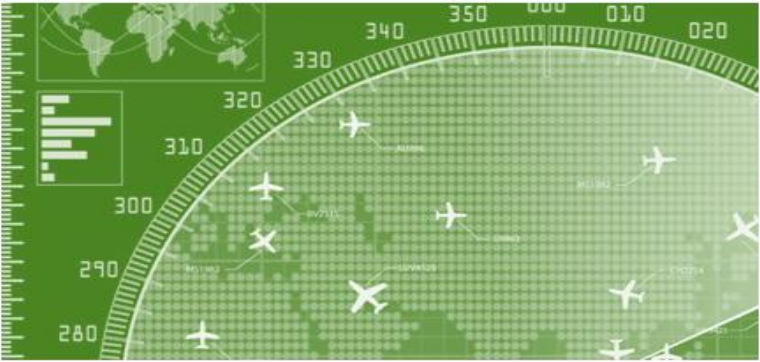At a given instance, ATC finds that the angle of elevation of an airplane from a point on the ground is 60o. After a flight of 30 seconds, it is observed that the angle of elevation changes to 30o. The height of the plane remains constantly as {tex}3000 \sqrt{3}{/tex} m. Use the above information to answer the questions that follow-

1. Draw a neat labelled figure to show the above situation diagrammatically.
2. What is the distance travelled by the plane in 30 seconds?

OR

Keeping the height constant, during the above flight, it was observed that after 15({tex}\sqrt{3}{/tex} -1) seconds, the angle of elevation changed to 45o. How much is the distance travelled in that duration.

3. What is the speed of the plane in km/hr.

## Model Papers Class 10 Maths Basic 2023

CBSE Class 10
Mathematics Basic (Code No. 241)
(Sample Paper 2022-23)

Time Allowed: 3 Hours
Maximum Marks: 80
General Instructions:

1. This Question Paper has 5 Sections A, B, C, D, and E.
2. Section A has 20 Multiple Choice Questions (MCQs) carrying 1 mark each.
3. Section B has 5 Short Answer-I (SA-I) type questions carrying 2 marks each.
4. Section C has 6 Short Answer-II (SA-II) type questions carrying 3 marks each.
5. Section D has 4 Long Answer (LA) type questions carrying 5 marks each.
6. Section E has 3 Case Based integrated units of assessment (4 marks each) with sub-parts of the values of 1, 1 and 2 marks each respectively.
7. All Questions are compulsory. However, an internal choice in 2 Qs of 2 marks, 2 Qs of 3 marks and 2 Questions of 5 marks has been provided. An internal choice has been provided in the 2 marks questions of Section E.
8. Draw neat figures wherever required. Take {tex}\pi{/tex} =22/7 wherever required if not stated.

### Class 10 Maths Basic Section A

Section A consists of 20 questions of 1 mark each.

1. If two positive integers p and q can be expressed as p = ab2 and q = a3b; a, b being prime numbers, then LCM (p, q) is
1. ab
2. a2b2
3. a3b2
4. a3b3
2. What is the greatest possible speed at which a man can walk 52 km and 91 km in an exact number of hours?
1. 17 km/hours
2. 7 km/hours
3. 13 km/hours
4. 26 km/hours
3. If one zero of the quadratic polynomial x2 + 3x + k is 2, then the value of k is
1. 10
2. -10
3. 5
4. -5
4.  Graphically, the pair of equations given by
6x – 3y + 10 = 0
2x – y + 9 = 0
represents two lines which are

1. intersecting at exactly one point.
2. parallel.
3. coincident.
4. intersecting at exactly two points.
5. If the quadratic equation x2 + 4x + k = 0 has real and equal roots, then
1. k < 4
2. k > 4
3. k = 4
4. {tex}\geq{/tex} 4
6. The perimeter of a triangle with vertices (0, 4), (0, 0) and (3, 0) is
1. 5 units
2. 12 units
3. 11 units
4. (7 + {tex}\sqrt{5}{/tex}) units
7. If in triangles ABC and DEF, {tex}\frac{\mathrm{AB}}{\mathrm{DE}}=\frac{\mathrm{BC}}{\mathrm{FD}}{/tex} then they will be similar, when
1. {tex}\angle B=\angle E{/tex}
2. {tex}\angle A=\angle D{/tex}
3. {tex}\angle B=\angle D{/tex}
4. {tex}\angle A=\angle F{/tex}
8. In which ratio the y-axis divides the line segment joining the points (5, -6) and (-1, -4)?.
1. 1 : 5
2. 5 : 1
3. 1 : 1
4. 1 : 2
9. In the figure, if PA and PB are tangents to the circle with centre O such that {tex}\angle{/tex}APB = 50o, then {tex}\angle{/tex}OAB is equal to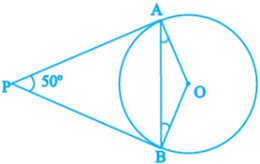1. 25o
2. 30o
3. 40o
4. 50o
10. If sin A = {tex}\frac{1}{2}{/tex}, then the value of sec A is:
1. {tex}\frac{\sqrt{3}}{2}{/tex}
2. {tex}\frac{1}{\sqrt{3}}{/tex}
3. {tex}\sqrt{3}{/tex}
4. 1
11. {tex}\sqrt{3}{/tex} cos2A + {tex}\sqrt{3}{/tex} sin2A is equal to
1. 1
2. {tex}\frac{1}{\sqrt{3}}{/tex}
3. {tex}\sqrt{3}{/tex}
4. 0
12.  The value of cos1o cos2o cos3o cos4o ________ cos90o is
1. 1
2. 0
3. -1
4. 2
13. If the perimeter of a circle is equal to that of a square, then the ratio of their areas is
1. 22 : 7
2. 14 : 11
3. 7 : 22
4. 11 : 14
14. If the radii of two circles are in the ratio of 4 : 3, then their areas are in the ratio of:
1. 4 : 3
2. 8 : 3
3. 16 : 9
4. 9 : 16
15. The total surface area of a solid hemisphere of radius 7 cm is:
1. 447{tex}\pi{/tex} cm2
2. 239{tex}\pi{/tex} cm2
3. 174{tex}\pi{/tex} cm2
4. 147{tex}\pi{/tex} cm2
16. For the following distribution:
 Class 0-5 5-10 10-15 15-20 20-25 Frequency 10 15 12 20 9

the upper limit of the modal class is

1. 10
2. 15
3. 20
4. 25
17. If the mean of the following distribution is 2.6, then the value of y is
 Variable(x) 1 2 3 4 5 Frequency 4 5 y 1 2
1. 3
2. 8
3. 13
4. 24
18. A card is selected at random from a well shuffled deck of 52 cards. The probability of its being a red face card is
1. {tex}\frac{3}{26}{/tex}
2. {tex}\frac{3}{13}{/tex}
3. {tex}\frac{2}{13}{/tex}
4. {tex}\frac{1}{2}{/tex}

Direction for questions 19 & 20: In question numbers 19 and 20, a statement of Assertion (A) is followed by a statement of Reason (R). Choose the correct option.

1. Assertion: If HCF of 510 and 92 is 2, then the LCM of 510 & 92 is 32460 Reason: as HCF(a,b) {tex}\times{/tex} LCM(a,b) = a {tex}\times{/tex} b
1. Both Assertion (A) and Reason (R) are true and Reason (R) is the correct explanation of Assertion (A).
2. Both Assertion (A) and Reason (R) are true but Reason (R) is not the correct explanation of Assertion (A).
3. Assertion (A) is true but Reason (R) is false.
4. Assertion (A) is false but Reason (R) is true.
2. Assertion (A): The ratio in which the line segment joining (2, -3) and (5, 6) internally divided by x axis is 1 : 2.
Reason (R): as formula for the internal division is {tex}\left(\frac{m x_2+n x_1}{m+n}, \frac{m y_2+n y_1}{m+n}\right){/tex}

1. Both Assertion (A) and Reason (R) are true and Reason (R) is the correct explanation of Assertion (A).
2. Both Assertion (A) and Reason (R) are true but Reason (R) is not the correct explanation of Assertion (A).
3. Assertion (A) is true but Reason (R) is false.
4. Assertion (A) is false but Reason (R) is true.

### Class 10 Maths Basic Section B

Section B consists of 5 questions of 2 marks each.

1. For what values of k will the following pair of linear equations have infinitely many solutions?
kx + 3y – (k – 3) = 0
12x + ky – k = 0
2. In the figure, altitudes AD and CE of {tex}\triangle{/tex}ABC intersect each other at the point P. Show that: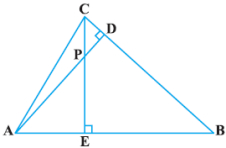1. {tex}\triangle \mathrm{ABD} \sim \triangle \mathrm{CBE}{/tex}
2. {tex}\triangle \mathrm{PDC} \sim \triangle \mathrm{BEC}{/tex}

OR

In the figure, DE || AC and DF || AE. Prove that {tex}\frac{\mathrm{BF}}{\mathrm{FE}}=\frac{\mathrm{BE}}{\mathrm{EC}}{/tex}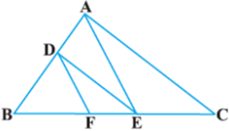3. Two concentric circles are of radii 5 cm and 3 cm. Find the length of the chord of the larger circle which touches the smaller circle.
4. If cot {tex}\theta=\frac{7}{8}{/tex}, evaluate {tex}\frac{(1+\sin \theta)(1-\sin \theta)}{(1+\cos \theta)(1-\cos \theta)}{/tex}
5. Find the perimeter of a quadrant of a circle of radius 14 cm.

OR

Find the diameter of a circle whose area is equal to the sum of the areas of the two circles of radii 24 cm and 7 cm.

### Class 10 Maths Basic Section C

Section C consists of 6 questions of 3 marks each.

1. Prove that {tex}\sqrt 5{/tex} is an irrational number.
2. Find the zeroes of the quadratic polynomial 6x2 – 3 – 7x and verify the relationship between the zeroes and the coefficients.
3. A shopkeeper gives books on rent for reading. She takes a fixed charge for the first two days, and an additional charge for each day thereafter. Latika paid ₹ 22 for a book kept for six days, while Anand paid ₹ 16 for the book kept for four days. Find the fixed charges and the charge for each extra day.

OR

Places A and B are 100 km apart on a highway. One car starts from A and another from B at the same time. If the cars travel in the same direction at different speeds, they meet in 5 hours. If they travel towards each other, they meet in 1 hour. What are the speeds of the two cars?

4. In the figure, PQ is a chord of length 8 cm of a circle of radius 5 cm. The tangents at P and Q intersect at a point T. Find the length TP.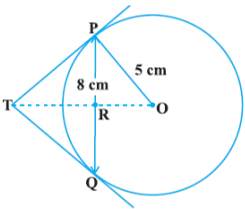5. Prove that
{tex}\frac{\tan \theta}{1-\cot \theta}+\frac{\cot \theta}{1-\tan \theta}{/tex} = 1 + sec{tex}\theta{/tex} cosec{tex}\theta{/tex}

OR

If sin {tex}\theta{/tex} + cos {tex}\theta{/tex} = {tex}\sqrt 3{/tex}, then prove that tan {tex}\theta{/tex} + cot {tex}\theta{/tex} = 1

6. Two dice are thrown at the same time. What is the probability that the sum of the two numbers appearing on the top of the dice is
1. 8?
2. 13?
3. less than or equal to 12?

### Class 10 Maths Basic Section D

Section D consists of 4 questions of 5 marks each.

1. An express train takes 1 hour less than a passenger train to travel 132 km between Mysore and Bangalore (without taking into consideration the time they stop at intermediate stations). If the average speed of the express train is 11km/h more than that of the passenger train, find the average speed of the two trains.

OR

A motor boat whose speed is 18 km/h in still water takes 1 hour more to go 24 km upstream than to return downstream to the same spot. Find the speed of the stream.

2. Prove that If a line is drawn parallel to one side of a triangle to intersect the other two sides in distinct points, the other two sides are divided in the same ratio. In the figure, find EC if {tex}\frac{\mathrm{AD}}{\mathrm{DB}}=\frac{\mathrm{AE}}{\mathrm{EC}}{/tex} using the above theorem.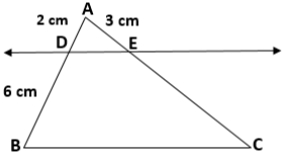3. A pen stand made of wood is in the shape of a cuboid with four conical depressions to hold pens. The dimensions of the cuboid are 15 cm by 10 cm by 3.5 cm. The radius of each of the depressions is 0.5 cm and the depth is 1.4 cm. Find the volume of wood in the entire stand.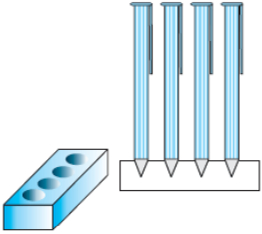OR

Ramesh made a bird-bath for his garden in the shape of a cylinder with a hemispherical depression at one end. The height of the cylinder is 1.45 m and its radius is 30 cm. Find the total surface area of the bird-bath.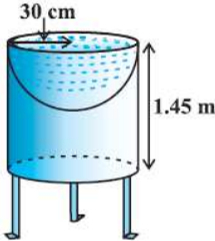4. A life insurance agent found the following data for distribution of ages of 100 policy holders. Calculate the median age, if policies are given only to persons having age 18 years onwards but less than 60 years.
 Age (in years) Number of policy holders Below 20 2 20-25 4 25-30 18 30-35 21 35-40 33 40-45 11 45-50 3 50-55 6 55-60 2

### Class 10 Maths Basic Section E

Case study based questions are compulsory.

1. Case Study – 1
In the month of April to June 2022, the exports of passenger cars from India increased by 26% in the corresponding quarter of 2021–22, as per a report. A car manufacturing company planned to produce 1800 cars in 4th year and 2600 cars in 8th year. Assuming that the production increases uniformly by a fixed number every year.Based on the above information answer the following questions.

1. Find the production in the 1st year.
2. Find the production in the 12th year.
3. Find the total production in first 10 years.

OR

In which year the total production will reach to 15000 cars?

2. Case Study – 2
In a GPS, The lines that run east-west are known as lines of latitude, and the lines running north-south are known as lines of longitude. The latitude and the longitude of a place are its coordinates and the distance formula is used to find the distance between two places. The distance between two parallel lines is approximately 150 km. A family from Uttar Pradesh planned a round trip from Lucknow (L) to Puri (P) via Bhuj (B) and Nashik (N) as shown in the given figure below.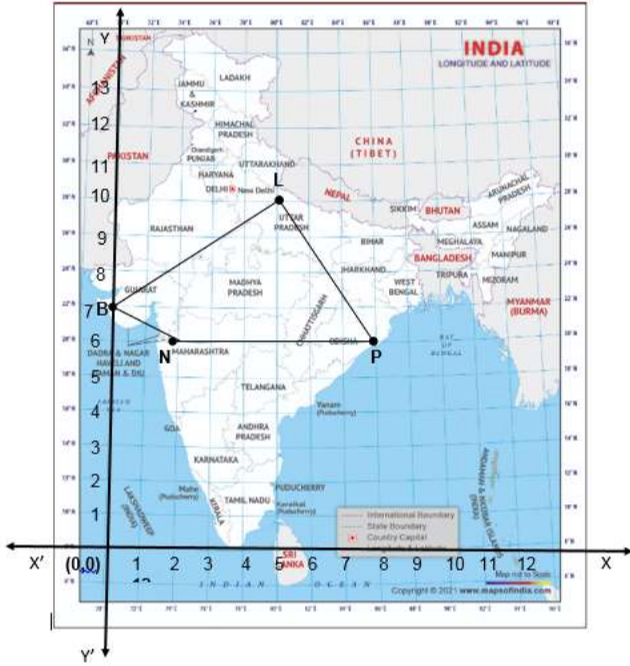Based on the above information answer the following questions using the coordinate geometry.

1. Find the distance between Lucknow (L) to Bhuj(B).
2. If Kota (K), internally divide the line segment joining Lucknow (L) to Bhuj (B) into 3 : 2 then find the coordinate of Kota (K).
3. Name the type of triangle formed by the places Lucknow (L), Nashik (N) and Puri (P)

OR

Find a place (point) on the longitude (y-axis) which is equidistant from the points Lucknow (L) and Puri (P).

3. Case Study – 3
Lakshaman Jhula is located 5 kilometers north-east of the city of Rishikesh in the Indian state of Uttarakhand. The bridge connects the villages of Tapovan to Jonk. Tapovan is in Tehri Garhwal district, on the west bank of the river, while Jonk is in Pauri Garhwal district, on the east bank. Lakshman Jhula is a pedestrian bridge also used by motorbikes. It is a landmark of Rishikesh.
A group of Class X students visited Rishikesh in Uttarakhand on a trip. They observed from a point (P) on a river bridge that the angles of depression of opposite banks of the river are 60o and 30o respectively. The height of the bridge is about 18 meters from the river.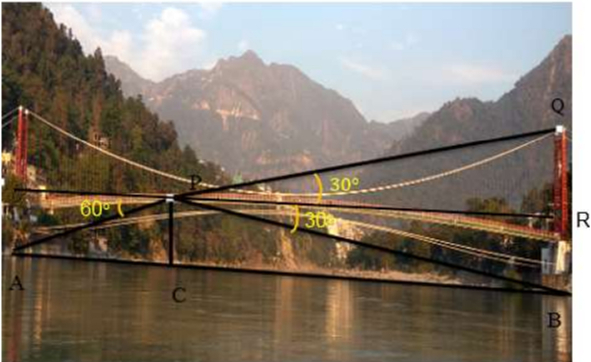Based on the above information answer the following questions.

1. Find the distance PA.
2. Find the distance PB
3. Find the width AB of the river.

OR

Find the height BQ if the angle of the elevation from P to Q be 30o.

These are questions only. To view and download the complete question paper with solutions install the myCBSEguide App from the google play store or login into our student dashboard.

## Sample Papers for Class 10 2022-23 in PDF

To download Sample Papers Class 10 Mathematics 2023, Science-Social Science, English Communicative, English Language and literature, Hindi Course A  and Hindi course B do check myCBSEguide app or website. myCBSEguide provides sample papers with solution, test papers for chapter-wise practice, NCERT solutions, NCERT Exemplar solutions, quick revision notes for ready reference, CBSE guess papers and CBSE important question papers. Sample Paper all are made available through the best app for CBSE students and myCBSEguide website.Test Generator

Create question paper PDF and online tests with your own name & logo in minutes.myCBSEguide

Question Bank, Mock Tests, Exam Papers, NCERT Solutions, Sample Papers, Notes

### 18 thoughts on “Class 10 Maths Sample Papers 2022-23”

1. Nice

2. It is better for cbse student

3. thanks.

4. Thanks for giving CBSE sample paper for students

5. can i get solution of this sample paper please as fast as possible

6. Thankyou very much?

7. thanks for your helping hand

8. Thanks
I clear some problems

9. Thqu for the paper these help a lot in preparing for examinations, and answers sheets provide an idea for giving answers.

10. thank u for your help.